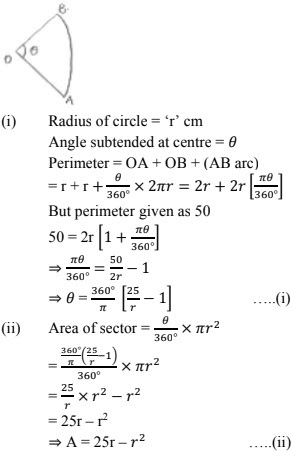# Chapter 15 Areas Related to Circles RD Sharma Solutions Exercise 15.2 Class 10 Maths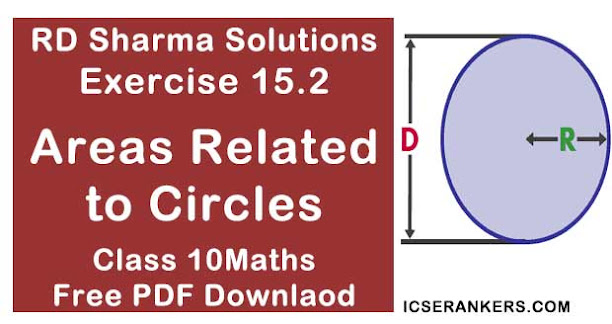Chapter Name RD Sharma Chapter 15 Areas Related to Circles Book Name RD Sharma Mathematics for Class 10 Other Exercises Exercise 15.1Exercise 15.3Exercise 15.4 Related Study NCERT Solutions for Class 10 Maths

### Exercise 15.2 Solutions

1. Find in terms of x the length of the arc that subtends an angle of 30° , at the centre of circle of radius 4 cm.

Solution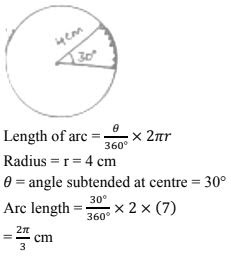2. Find the angle subtended at the centre of circle of radius 5cm by an arc of length (5π/3) cm.

Solution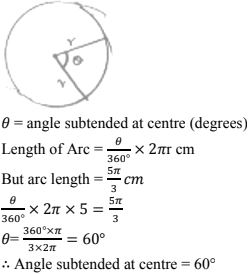3. An arc of length 20π cm subtends an angle of 144° at centre of circle. Find the radius of the circle.
Solution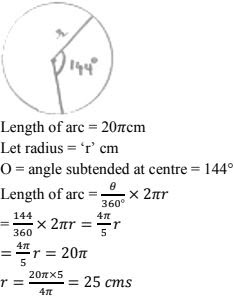4. An arc of length 15cm subtends an angle of 45° at the centre of a circle. Find in terms of π, radius of the circle.
Solution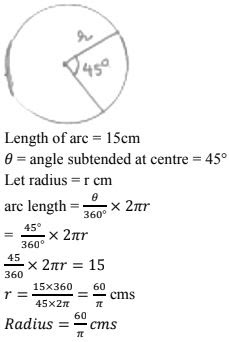5. Find the angle subtended at the centre of circle of radius  'a' cm by an arc of length aπ/4 cm
Solution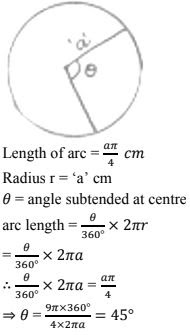6. A sector of circle of radius 4cm contains an angle of 30° . Find the area of sector.
Solution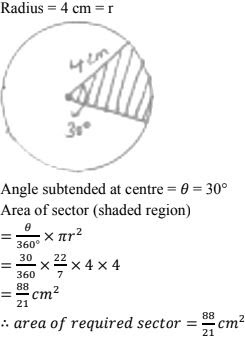7. A sector of a circle of radius 8cm contains the angle of 135° . Find the area of sector.
Solution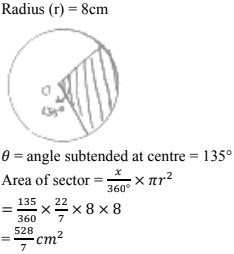8. The area of sector of circle of radius 2cm is πcm2. Find the angle contained by the sector.
Solution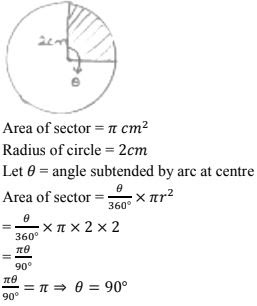9. The area of sector of circle of radius 5cm is 5πcm2 . Find the angle contained by the sector.
Solution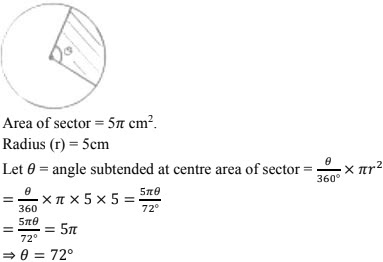10. AB is a chord or circle with centre O and radius 4cm. AB is length of 4cm. Find the area of sector of the circle formed by chord AB
Solution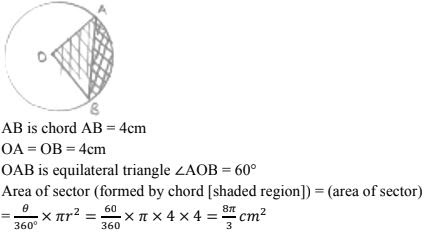11. In a circle of radius 35cm, an arc subtends an angle of 72° at the centre. Find the length of arc and area of sector.
Solution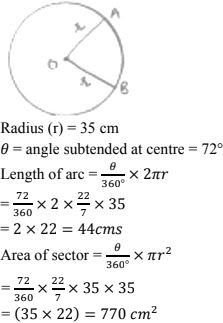12. The perimeter of a sector of circle of radius 5.7m is 27.2m. Find the area of sector.
Solution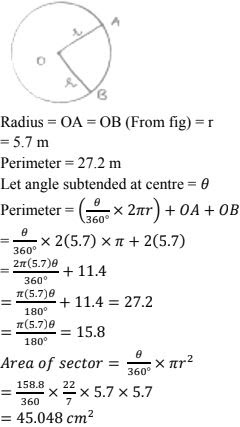13. The perimeter of certain sector of circle of radius 5.6 m is 27.2 m. Find the area of sector.
Solution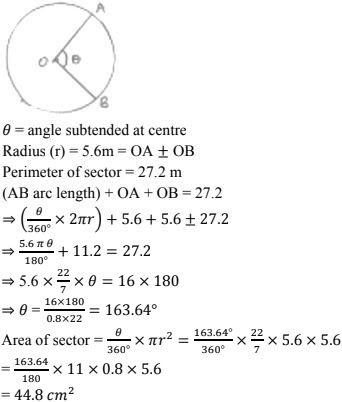14. A sector is cut - off from a circle of radius 21 cm the angle of sector is 120°. Find the length of its arc and its area.
Solution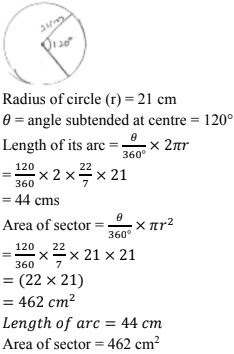15. The minute hand of a clock is √21 cm long. Find area described by the minute hand on the face of clock between 7am and 7:05 am
Solution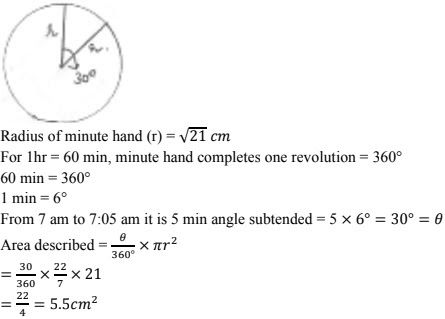16. The minute hand of clock is 10cm long. Find the area of the face of the clock described by the minute hand between 8 am and 8:25 am
Solution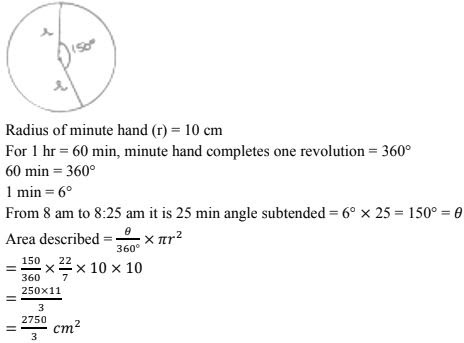17. A sector of 56° cut out from a circle contains area of 4.4 cm2 . Find the radius of the circle
Solution
Angle subtended by sector at centre θ = 56°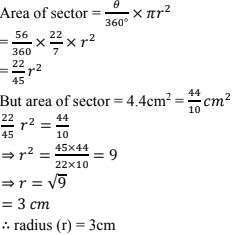18. In circle of radius 6cm, chord of length 10cm makes an angle of 110° at the centre of circle find
(i) Circumference of the circle
(ii) Area of the circle
(iii) Length of arc
(iv) The area of sector
Solution
(i) Radius of circle (r) = 6cm
Angle subtended at the centre = 110°
Circumference of the circle = 2πr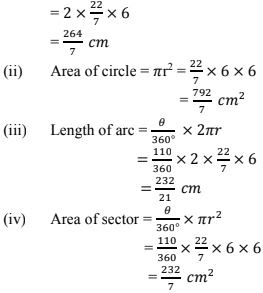19. Below fig. shows a sector of a circle, centre O containing an angle θ° Prove that
(i) Perimeter of shaded region is r(tan θ + sec θ + πθ/180 - 1)
(ii) Area of shaded region is r2(tan θ - πθ/180)
Solution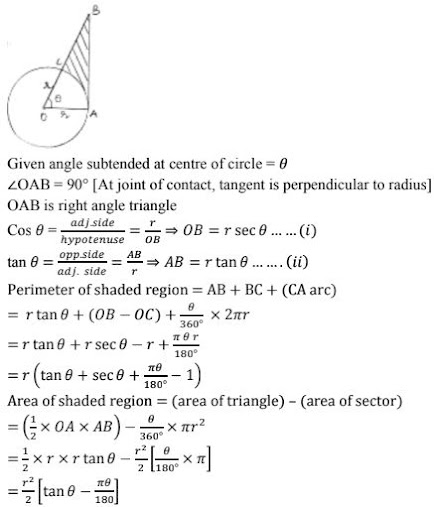20. The diagram shows a sector of circle of radius 'r' can containing an angle θ. The area of  sector is A cm2 and perimeter of sector is 50 cm. Prove that
(i) θ = 360/π(25/r - 1)
(ii) A = 25r - r2.
Solution